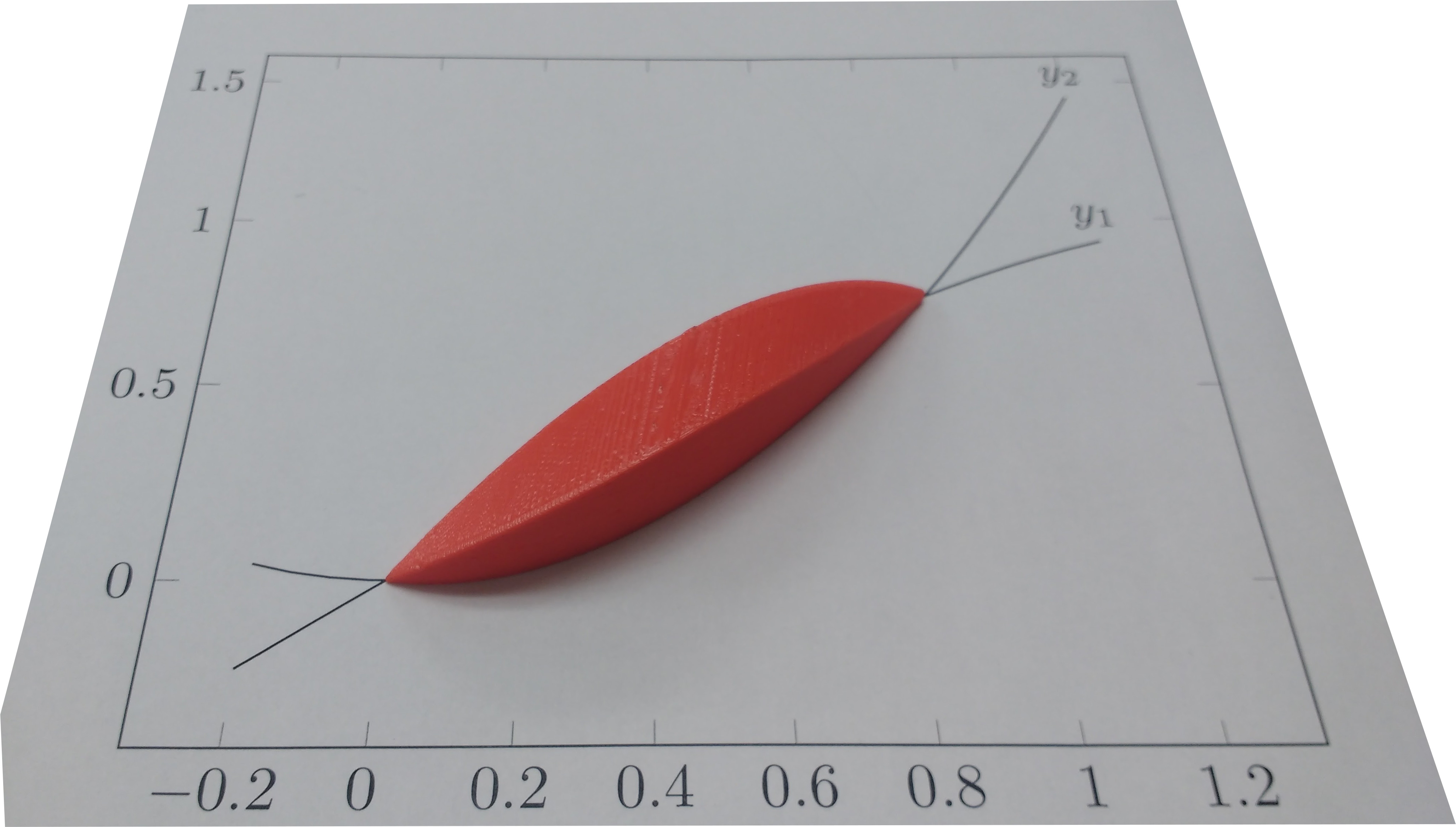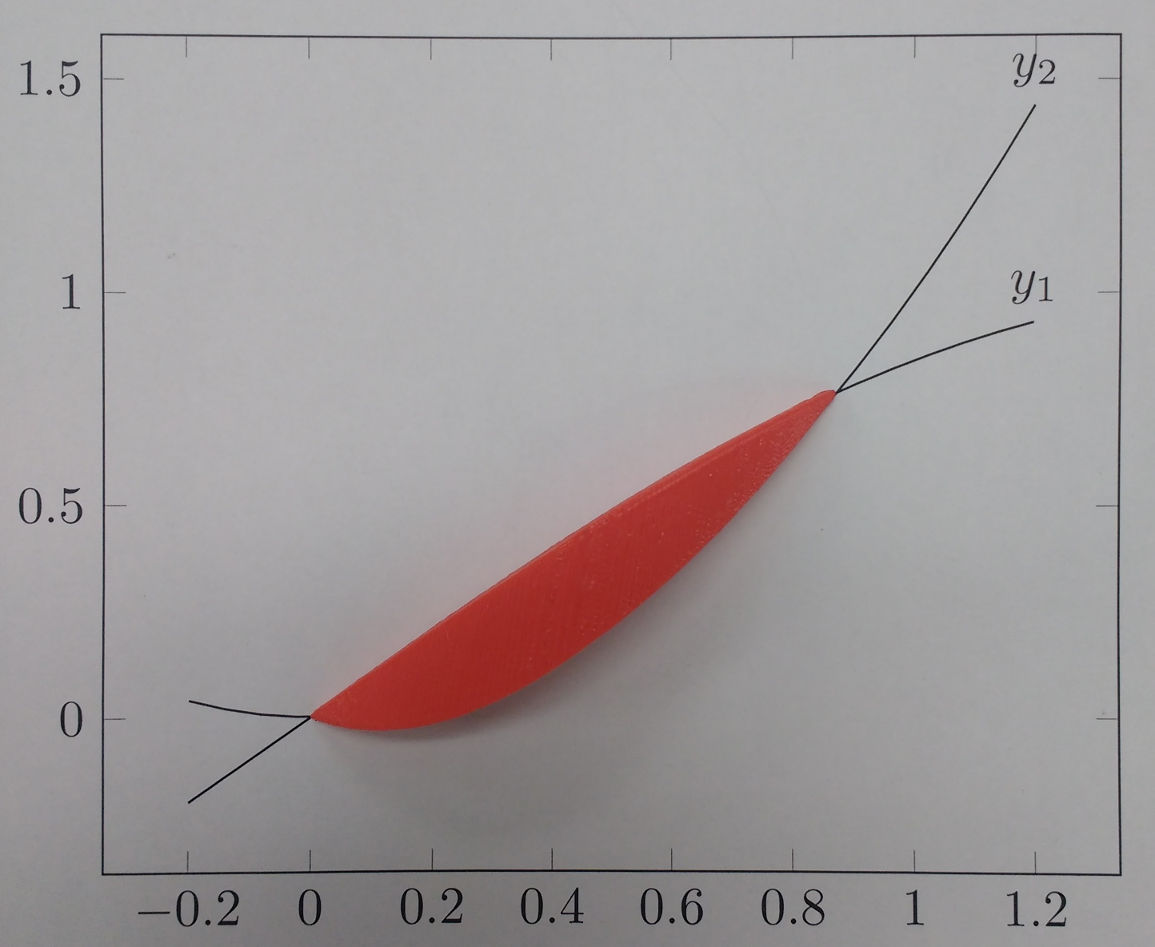#!/usr/bin/env python # This post was written as literate Python. # --- # title: Rendering a 3D shape with Python # date: 2016-11-09 # tags: ["Python", "OpenSCAD", "3D Printing", "Algorithm"] # slug: python-3d-rendering # --- # A friend wanted to 3D-print a shape to demonstrate using calculus to find the volume of solids of known cross-section. # The shape he wanted was a graph of $sin(x)$ vs $x^2$, # where each vertical slice of the intersection was a square. # Here's the graph, with $y_1 = sin(x)$ and $y_2 = x^2$. The blue lines show the edge of each square. # He couldn't figure out how to do this in a CAD program (I'm not even sure if it's possible), # so he asked me if I could write some code to render it. # # The first attempt I made was written directly in [OpenSCAD](http://www.openscad.org/); # I wrote a small loop to union together a collection of cube() calls, one for each slice. # This had several problems, however. # First, because I was using cubes it looked very chunky unless the slices were very small. # Second, I ran into [a bug in OpenSCAD](https://github.com/openscad/openscad/issues/350) # which causes something like $O(n^2)$ performance for this scenario # and the file took about 2 hours to render. # Finally, the STL file it emitted was 1.8 MB and crashed the slicer. # Clearly, this solution was not going to work. # Instead, I wrote a python script to output an OpenSCAD file containing a single polyhedron, # which I could then render into an STL file and hand off to him to slice and print. # In addition to not crashing the slicer, # this approach also had the advantage of allowing the resolution to be much coarser # while still avoiding the 'stair-step' problem of the original multiple cube() approach. import math import scipy # ## Setting up # First, the formulae: def y1(x): return math.sin(x) def y2(x): return x*x # $sin(x) = x^2$ is true for $x = 0$ and $x \approx 0.876$, so that's where I'll start and stop. START = 0 END = 0.876726 # I'll draw 50 slices, and scale everything up by 30. SLICES = 50 SCALE = 30 # OpenSCAD's [polyhedron() function](https://en.wikibooks.org/wiki/OpenSCAD_User_Manual/Primitive_Solids#polyhedron) # takes two arguments, points and triangles. # (Versions 2014.03 and later can also take faces instead of triangles, but I'm still using 2014.01.) # points is a list of $[x, y, z]$ triplets, and triangles then indexes into the list of points to build the triangles. # I therefore make a function point(x, y, z) # which will save the point into the list and return the index into this list. # Most of the program then works using these indexes, rather than the actual point coordinates. points = [] def point(x, y, z): points.append( (x*SCALE,y*SCALE,z*SCALE) ) return len(points)-1 # ## Slicing the shape # The first step is to slice the shape and get a list of squares. # (OpenSCAD expects the points on a triangle to be clockwise when looking at them from the outside, # so throughout the program I maintain this clockwise orientation.) Here's the square; line $\overline{P_2 P_3}$ is the part of the square that sits on the graph. The remainder of the square sits above it. # I slice the shape into SLICES slices, and output the above square for each slice. # This is also where I convert the coordinates into point-list indexes as explained above. def get_slices(): for x in scipy.arange(START, END, (END-START)/SLICES): yield ( point(x, y1(x), y1(x)-y2(x)), point(x, y2(x), y1(x)-y2(x)), point(x, y2(x), 0), point(x, y1(x), 0), ) # ## Building the polyhedron # All of these slices I have are *inside* the shape, # which isn't the part that's visible. # The next step is to get all of the faces of the polyhedron # by connecting the edge of adjoining slices into quadrilaterals. def get_faces(): slices = list(get_slices()) prev = slices # First, I make an "end cap" using the slice at the start of the shape. # This is necessary because otherwise the end will be left 'open' if the first slice is not of size 0, # and the shape [cannot be exported](https://en.wikibooks.org/wiki/OpenSCAD_User_Manual/STL_Import_and_Export#STL_Export_2) # because it is not a valid 2-manifold. yield slices # Next I generate quadrilaterals conecting each of the sides of ajoining slices. # Doing this is relatively simple: #
$$# \mbox{Given slices} # \substack { # \square T_0 T_1 T_2 T_3 \\ # \square P_0 P_1 P_2 P_3 # } # \mbox{, emit faces} # \substack { # \square T_0 P_0 P_1 T_1 \\ # \square T_1 P_1 P_2 T_2 \\ # \square T_2 P_2 P_3 T_3 \\ # \square T_3 P_3 P_0 T_0 # }. #$$
# (Notice that they all go clockwise when facing outward.) for this in slices[1:]: def face(a, b): return ( this[a], prev[a], prev[b], this[b], ) yield face(0, 1) yield face(1, 2) yield face(2, 3) yield face(3, 0) prev = this # The last step in making the polyhedron is to cap off the back end the same as I did with the front end (see above). yield prev # ## Convert quadrilaterals into triangles # I now have a list of faces for the polyhedron. For newer versions of OpenSCAD I could just pass this list in to the polyhedron() function; unfortunately as I mentioned before my version of OpenSCAD only accepts a list of triangles. # Therefore, I take each face (a quadrilateral $\square F_0 F_1 F_2 F_3$) and divide it into two triangles, $\triangle F_0 F_1 F_3$ and $\triangle F_1 F_2 F_3$. (Again, I'm being very careful to keep them in clockwise order.) def get_triangles(): for face in get_faces(): yield (face, face, face) yield (face, face, face) # ## Write the file # Finally, I print out the OpenSCAD file, which simply consists of a single call to polyhedron(): print "polyhedron(" print " convexity = 1," print " triangles = [" for triangle in get_triangles(): print " [{0}, {1}, {2}],".format(*triangle) print " ]," print " points = [" for point in points: print " [{0}, {1}, {2}],".format(*point) print " ]" print ");" # And finally, here's what the shape looks like when it's printed out. # In addition to the Python program linked at the top of this post, # you can also download # [the OpenSCAD file](shape.scad) and # [the STL file](shape.stl). ##